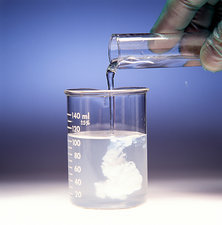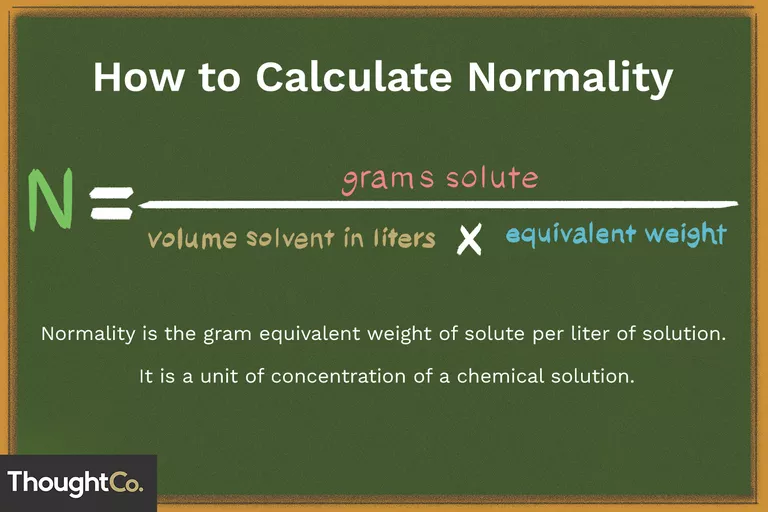# Normality Study Guide

Introduction

The precipitate of a white solid, silver chloride, is produced when aqueous silver nitrate is introduced to a solution containing potassium chloride. When two soluble salt solutions are mixed, a precipitation process occurs, resulting in the production of an insoluble salt. The precipitate is the insoluble salt that separates from the solution. But what if we wanted to understand precisely how much precipitate we will obtain from the reaction? In precipitation reactions, normality is used to calculate the number of ions that are expected to precipitate.Source

## WHAT IS NORMALITY IN CHEMISTRY?

• Measurement of concentration corresponding to the gram equivalent weight per liter of solution is called normality.
• The reactive capacity of a chemical is measured in grams equivalent weight.
• The normality of a solution is determined by the involvement of the solute in the reaction.
• N, eq/L, and meq/L are standard units of normality.
• The corresponding concentration of a solution is also termed normality.Source

## NORMALITY FORMULA

Normality = Number of gram equivalents × (volume of solution in litres)-1

Number of gram equivalents = weight of solute × (Equivalent weight of solute)-1

N = Molarity × Molar mass × (Equivalent mass)-1

N = Molarity × Basicity = Molarity × Acidity

## HOW TO CALCULATE NORMALITY

• Gathering information regarding the equivalent weight of the reactive substance or the solute is the first strategy that students may use.
• The number of gram equivalents of the solute is calculated in the second phase.
• The volume is measured in liters.
• Finally, using the formula and changing the values, normality is computed.

## USES OF NORMALITY

• In acid-base chemical reactions, establishing concentrations. Normality, for example, is used to show the quantities of hydronium ions (H3O ) or hydroxide ions (OH–) in a solution.
• In precipitation reactions, normality is used to calculate the number of ions that are expected to precipitate in a given reaction.
• It’s utilized in redox processes to determine how many electrons a reducing or oxidizing substance can take or contribute.

# Conclusion

• Measurement of concentration corresponding to the gram equivalent weight per liter of solution is called normality.
• N, eq/L, and meq/L are standard units of normality.
• N = Molarity × Molar mass × (Equivalent mass)-1

## FAQs:

1. What is normality in chemistry, for example?

A normal is one gram of a solute equivalent per liter of solution. Because hydrochloric acid is a powerful acid that fully dissociates in water, a 1 N HCl solution will indeed be 1 N for H or Cl- ions in acid-base interactions.

2. What is the difference between molarity and normality?

The major distinction between molarity and normalcy is that molarity refers to the number of moles of a chemical existing in a mixture, whereas normality refers to the number of gram equivalents of a compound present in a combination.

3. Why do we use normality?

In precipitation reactions, normality is used to calculate the number of ions that are expected to precipitate in a given reaction. It’s utilized in redox processes to figure out how many electrons a reducing or oxidizing substance can take or contribute.

We hope you enjoyed studying this lesson and learned something cool about Normality! Join our Discord community to get any questions you may have answered and to engage with other students just like you! Don’t forget to download our App to experience our fun VR classrooms – we promise, it makes studying much more fun! 😎

## SOURCES

1. Normality: https://byjus.com/ accessed 12 march 2022
2. How to Calculate Normality: https://www.thoughtco.com/how-to-calculate-normality-609580 accessed 12 march 2022
3. Definition of Normality: https://www.thoughtco.com/definition-of-normality-in-chemistry-605419 accessed 12 march 2022
]]>